Skip to main content Accessibility help
Home
Hostname: page-component-747cfc64b6-7hjq6 Total loading time: 0.249 Render date: 2021-06-13T15:44:36.676Z Has data issue: true Feature Flags: { "shouldUseShareProductTool": true, "shouldUseHypothesis": true, "isUnsiloEnabled": true, "metricsAbstractViews": false, "figures": true, "newCiteModal": false, "newCitedByModal": true, "newEcommerce": true }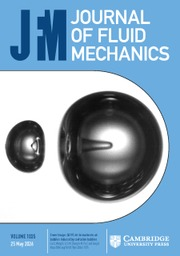Journal of Fluid Mechanics

# Effects of Reynolds number and Stokes number on particle-pair relative velocity in isotropic turbulence: a systematic experimental study

Published online by Cambridge University Press:  26 January 2018

Corresponding
E-mail address:

## Abstract

The effects of Reynolds number (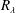$R_{\unicode[STIX]{x1D706}}$ ) and Stokes number (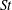$St$ ) on particle-pair relative velocity (RV) are investigated systematically using a recently developed planar four-frame particle tracking technique in a novel homogeneous and isotropic turbulence chamber. We compare the measured results with direct numerical simulation (DNS), verifying whether the conclusions of the DNS for simplified conditions and limited$R_{\unicode[STIX]{x1D706}}$ are still valid in reality. Two experiments are performed: varying$R_{\unicode[STIX]{x1D706}}$ between 246 and 357 at six$St$ values, and varying$St$ between 0.02 and 4.63 at five$R_{\unicode[STIX]{x1D706}}$ values. The measured mean inward particle-pair RV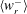$\langle w_{r}^{-}\rangle$ as a function of separation distance$r$ is compared with the DNS under closely matched conditions. At all experimental conditions, an excellent agreement is achieved, except when the particle separation distance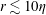$r\lesssim 10\unicode[STIX]{x1D702}$ ($\unicode[STIX]{x1D702}$ is the Kolmogorov length scale), where the experimental$\langle w_{r}^{-}\rangle$ is consistently higher, possibly due to particle polydispersity and finite laser thickness in the experiments (Dou et al., arXiv:1712.07506, 2017). At any fixed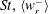$St,\langle w_{r}^{-}\rangle$ is essentially independent of$R_{\unicode[STIX]{x1D706}}$ , echoing the DNS finding of Ireland et al. (J. Fluid Mech., vol. 796, 2016, pp. 617–658). At any fixed$R_{\unicode[STIX]{x1D706}}$ ,$\langle w_{r}^{-}\rangle$ increases with$St$ at small$r$ , showing dominance of the path-history effect in the dissipation range when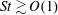$St\gtrsim O(1)$ , but decreases with$St$ at large$r$ , indicating dominance of inertial filtering. We further compare the$\langle w_{r}^{-}\rangle$ and RV variance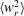$\langle w_{r}^{2}\rangle$ from experiments with DNS and theoretical predictions by Pan & Padoan (J. Fluid Mech., vol. 661, 2010, pp. 73–107). For$St\lesssim 1$ , experimental$\langle w_{r}^{-}\rangle$ and$\langle w_{r}^{2}\rangle$ match these values well at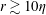$r\gtrsim 10\unicode[STIX]{x1D702}$ , but they are higher than both DNS and theory at$r\lesssim 10\unicode[STIX]{x1D702}$ . For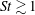$St\gtrsim 1$ ,$\langle w_{r}^{-}\rangle$ from all three match well, except for$r\lesssim 10\unicode[STIX]{x1D702}$ , for which experimental values are higher, while$\langle w_{r}^{2}\rangle$ from experiment and DNS are much higher than theoretical predictions. We discuss potential causes of these discrepancies. What this study shows is the first experimental validation of$R_{\unicode[STIX]{x1D706}}$ and$St$ effect on inertial particle-pair$\langle w_{r}^{-}\rangle$ in homogeneous and isotropic turbulence.

## JFM classification

Type
JFM Papers
Information
Journal of Fluid Mechanics , 25 March 2018 , pp. 271 - 292
Copyright
© 2018 Cambridge University Press

## Access options

Get access to the full version of this content by using one of the access options below.

## Footnotes

Present address: 319 Latrobe Hall, Johns Hopkins University, Baltimore, MD 21218, USA

## References

Ayala, O., Rosa, B., Wang, L.-P. & Grabowski, W. W. 2008 Effects of turbulence on the geometric collision rate of sedimenting droplets. Part 1. Results from direct numerical simulation. New J. Phys. 10, 075015.CrossRefGoogle Scholar
Ayyalasomayajula, S., Warhaft, Z. & Collins, L. R. 2008 Modeling inertial particle acceleration statistics in isotropic turbulence. Phys. Fluids 20, 095104.CrossRefGoogle Scholar
Bec, J., Biferale, L., Boffetta, G., Celani, A., Cencini, M., Lanotte, A., Musacchio, S. & Toschi, F. 2006 Acceleration statistics of heavy particles in turbulence. J. Fluid Mech. 550, 349358.CrossRefGoogle Scholar
Bec, J., Biferale, L., Lanotte, A. S., Scagliarini, A. & Toschi, F. 2010 Turbulent pair dispersion of inertial particles. J. Fluid Mech. 645, 497528.CrossRefGoogle Scholar
Bragg, A. D. & Collins, L. R. 2014a New insights from comparing statistical theories for inertial particles in turbulence: I. Spatial distribution of particles. New J. Phys. 16, 055013.Google Scholar
Bragg, A. D. & Collins, L. R. 2014b New insights from comparing statistical theories for inertial particles in turbulence: II. Relative velocities. New J. Phys. 16, 055014.Google Scholar
Bragg, A. D., Ireland, P. J. & Collins, L. R. 2015a Mechanisms for the clustering of inertial particles in the inertial range of isotropic turbulence. Phys. Rev. E 92, 023029.Google ScholarPubMed
Bragg, A. D., Ireland, P. J. & Collins, L. R. 2015b On the relationship between the non-local clustering mechanism and preferential concentration. J. Fluid Mech. 780, 327343.CrossRefGoogle Scholar
Bragg, A. D., Ireland, P. J. & Collins, L. R. 2016 Forward and backward in time dispersion of fluid and inertial particles in isotropic turbulence. Phys. Fluids 28, 013305.CrossRefGoogle Scholar
Collins, L. R. & Keswani, A. 2004 Reynolds number scaling of particle clustering in turbulent aerosols. New J. Phys. 6, 119.CrossRefGoogle Scholar
Cuzzi, J. N., Hogan, R. C., Paque, J. M. & Dobrovolskis, A. R. 2001 Size-selective concentration of chondrules and other small particles in protoplanetary nebula turbulence. Astrophys. J. 546, 496.CrossRefGoogle Scholar
Dou, Z.2017 Experimental study of inertial particle-pair relative velocity in isotropic turbulence (order no. 10255106), Mechanical and Aerospace Engineering. University at Buffalo – SUNY, Buffalo, NY.Google Scholar
Dou, Z., Ireland, P. J., Bragg, A. D., Liang, Z., Collins, L. R. & Meng, H.2017 Particle-pair relative velocity measurement in high-Reynolds-number homogeneous and isotropic turbulence using 4-frame particle tracking velocimetry, arXiv:1712.07506.Google Scholar
Dou, Z., Pecenak, Z. K., Cao, L., Woodward, S. H., Liang, Z. & Meng, H. 2016 PIV measurement of high-Reynolds-number homogeneous and isotropic turbulence in an enclosed flow apparatus with fan agitation. Meas. Sci. Technol. 27, 035305.CrossRefGoogle Scholar
Dullemond, C. & Dominik, C. 2005 Dust coagulation in protoplanetary disks: a rapid depletion of small grains. Astron. Astrophys. 434, 971986.CrossRefGoogle Scholar
Eaton, J. K. & Fessler, J. R. 1994 Preferential concentration of particles by turbulence. Intl J. Multiphase Flow 20, 169209.CrossRefGoogle Scholar
Falkovich, G., Fouxon, A. & Stepanov, M. G. 2002 Acceleration of rain initiation by cloud turbulence. Nature 419, 151154.CrossRefGoogle ScholarPubMed
Falkovich, G. & Pumir, A. 2007 Sling effect in collisions of water droplets in turbulent clouds. J. Atmos. Sci. 64, 44974505.CrossRefGoogle Scholar
Gustavsson, K. & Mehlig, B. 2011 Distribution of relative velocities in turbulent aerosols. Phys. Rev. E 84, 045304.Google ScholarPubMed
Ijzermans, R. H. A., Meneguz, E. & Reeks, M. W. 2010 Segregation of particles in incompressible random flows: singularities, intermittency and random uncorrelated motion. J. Fluid Mech. 653, 99136.CrossRefGoogle Scholar
Ireland, P. J., Bragg, A. D. & Collins, L. R. 2016a The effect of Reynolds number on inertial particle dynamics in isotropic turbulence. Part 1. Simulations without gravitational effects. J. Fluid Mech. 796, 617658.CrossRefGoogle Scholar
Ireland, P. M., Noda, M., Jarrett, E. D., Fujii, S., Nakamura, Y., Wanless, E. J. & Webber, G. B. 2016b Electrostatic formation of liquid marbles – influence of drop and particle size. Powder Technol. 303, 5558.CrossRefGoogle Scholar
de Jong, J., Salazar, J. P. L. C., Woodward, S. H., Collins, L. R. & Meng, H. 2010 Measurement of inertial particle clustering and relative velocity statistics in isotropic turbulence using holographic imaging. Intl J. Multiphase Flow 36, 324332.CrossRefGoogle Scholar
Laviéville, J., Deutsch, E. & Simonin, O.1995 Large eddy simulation of interactions between colliding particles and a homogeneous isotropic turbulence field. In ASME-Publications-Fed, vol. 228, pp. 347–358.Google Scholar
Laviéville, J., Simonin, O., Berlemont, A. & Chang, Z.1997 Validation of inter-particle collision models based on large eddy simulation in gas–solid turbulent homogeneous shear flow. In ASME FEDSM, pp. 22–26.Google Scholar
Pan, L. & Padoan, P. 2013 Turbulence-induced relative velocity of dust particles. I. Identical particles. Astrophys. J. 776, 12.CrossRefGoogle Scholar
Pan, L. B. & Padoan, P. 2010 Relative velocity of inertial particles in turbulent flows. J. Fluid Mech. 661, 73107.CrossRefGoogle Scholar
Rosa, B., Parishani, H., Ayala, O., Grabowski, W. W. & Wang, L.-P. 2013 Kinematic and dynamic collision statistics of cloud droplets from high-resolution simulations. New J. Phys. 15, 045032.CrossRefGoogle Scholar
Salazar, J. P. L. C. & Collins, L. R. 2012a Inertial particle acceleration statistics in turbulence: effects of filtering, biased sampling, and flow topology. Phys. Fluids 24, 083302.CrossRefGoogle Scholar
Salazar, J. P. L. C. & Collins, L. R. 2012b Inertial particle relative velocity statistics in homogeneous isotropic turbulence. J. Fluid Mech. 696, 4566.CrossRefGoogle Scholar
Salazar, J. P. L. C., De Jong, J., Cao, L. J., Woodward, S. H., Meng, H. & Collins, L. R. 2008 Experimental and numerical investigation of inertial particle clustering in isotropic turbulence. J. Fluid Mech. 600, 245256.CrossRefGoogle Scholar
Saw, E.-W., Bewley, G. P., Bodenschatz, E., Ray, S. S. & Bec, J. 2014 Extreme fluctuations of the relative velocities between droplets in turbulent airflow. Phys. Fluids 26, 111702.CrossRefGoogle Scholar
Shaw, R. A. 2003 Particle–turbulence interactions in atmospheric clouds. Annu. Rev. Fluid Mech. 35, 183227.CrossRefGoogle Scholar
Siebert, H., Lehmann, K. & Wendisch, M. 2006 Observations of small-scale turbulence and energy dissipation rates in the cloudy boundary layer. J. Atmos. Sci. 63, 14511466.CrossRefGoogle Scholar
Simonin, O.2000 Statistical and continuum modelling of turbulent reactive particulate flows. Lecture Series, vol. 6.Google Scholar
Sundaram, S. & Collins, L. R. 1997 Collision statistics in an isotropic particle-laden turbulent suspension. 1. Direct numerical simulations. J. Fluid Mech. 335, 75109.CrossRefGoogle Scholar
Wang, L. P., Wexler, A. S. & Zhou, Y. 2000 Statistical mechanical description and modelling of turbulent collision of inertial particles. J. Fluid Mech. 415, 117153.CrossRefGoogle Scholar
Wilkinson, M., Mehlig, B. & Bezuglyy, V. 2006 Caustic activation of rain showers. Phys. Rev. Lett. 97, 048501.CrossRefGoogle ScholarPubMed
Wu, W., Soligo, G., Marchioli, C., Soldati, A. & Piomelli, U. 2017 Particle resuspension by a periodically forced impinging jet. J. Fluid Mech. 820, 284311.CrossRefGoogle Scholar
Zaichik, L. I. & Alipchenkov, V. M. 2009 Statistical models for predicting pair dispersion and particle clustering in isotropic turbulence and their applications. New J. Phys. 11, 103018.CrossRefGoogle Scholar
Zaichik, L. I., Simonin, O. & Alipchenkov, V. M. 2006 Collision rates of bidisperse inertial particles in isotropic turbulence. Phys. Fluids 18, 035110.CrossRefGoogle Scholar
Zsom, A., Ormel, C. W., Guettler, C., Blum, J. & Dullemond, C. P. 2010 The outcome of protoplanetary dust growth: pebbles, boulders, or planetesimals? II. Introducing the bouncing barrier. Astron. Astrophys. 513, A57.CrossRefGoogle Scholar
10
Cited by

# Send article to Kindle

To send this article to your Kindle, first ensure no-reply@cambridge.org is added to your Approved Personal Document E-mail List under your Personal Document Settings on the Manage Your Content and Devices page of your Amazon account. Then enter the ‘name’ part of your Kindle email address below. Find out more about sending to your Kindle. Find out more about sending to your Kindle.

Note you can select to send to either the @free.kindle.com or @kindle.com variations. ‘@free.kindle.com’ emails are free but can only be sent to your device when it is connected to wi-fi. ‘@kindle.com’ emails can be delivered even when you are not connected to wi-fi, but note that service fees apply.

Find out more about the Kindle Personal Document Service.

Effects of Reynolds number and Stokes number on particle-pair relative velocity in isotropic turbulence: a systematic experimental study
Available formats
×

# Send article to Dropbox

To send this article to your Dropbox account, please select one or more formats and confirm that you agree to abide by our usage policies. If this is the first time you use this feature, you will be asked to authorise Cambridge Core to connect with your <service> account. Find out more about sending content to Dropbox.

Effects of Reynolds number and Stokes number on particle-pair relative velocity in isotropic turbulence: a systematic experimental study
Available formats
×

# Send article to Google Drive

To send this article to your Google Drive account, please select one or more formats and confirm that you agree to abide by our usage policies. If this is the first time you use this feature, you will be asked to authorise Cambridge Core to connect with your <service> account. Find out more about sending content to Google Drive.

Effects of Reynolds number and Stokes number on particle-pair relative velocity in isotropic turbulence: a systematic experimental study
Available formats
×
×

#### Reply to:Submit a response

Please enter your response.

#### Your details

Please enter a valid email address.

#### Conflicting interests

Do you have any conflicting interests? *